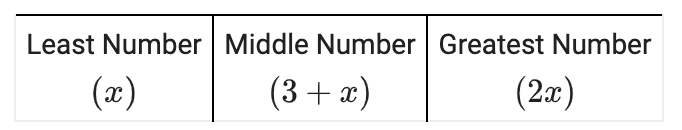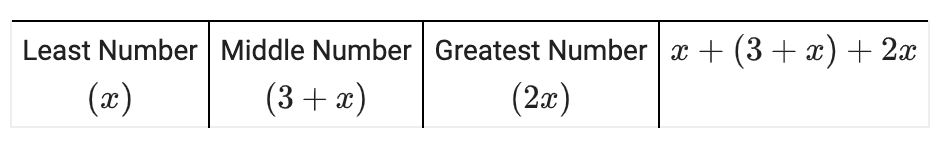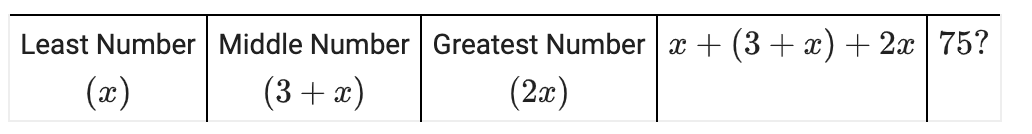### Home > CC3 > Chapter 1 > Lesson 1.1.3 > Problem1-20

1-20.

Solve the problem below by defining a variable and then writing an equation. If you find this too challenging, then use the $5$-D Process described in this lesson’s Math Notes box to help you get started. State your solution in a sentence.

Jabari is thinking of three numbers. The greatest number is twice as large as the least number. The middle number is three more than the least number. The sum of the three numbers is $75$. Find the numbers.

Rewrite the word problem as an equation or a system of equations.

Let the least number equal $x$.

A row with 3 columns labeled, left to right, as follows: Least number, x. Middle number, 3 + x. Greatest number, 2 x.

Fourth column added, labeled, x + (3 + x) + 2 x.Make a guess for what $x$ could be. Was your answer higher or lower than $75$? Adjust your next guess accordingly.